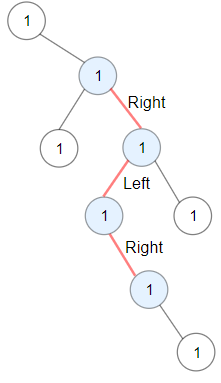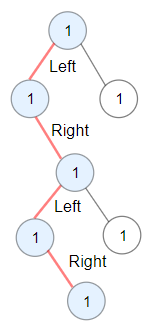# 1372. Longest ZigZag Path in a Binary Tree

You are given the `root` of a binary tree.

A ZigZag path for a binary tree is defined as follow:

• Choose any node in the binary tree and a direction (right or left).
• If the current direction is right, move to the right child of the current node; otherwise, move to the left child.
• Change the direction from right to left or from left to right.
• Repeat the second and third steps until you can’t move in the tree.

Zigzag length is defined as the number of nodes visited – 1. (A single node has a length of 0).

Return the longest ZigZag path contained in that tree.

Example 1:```Input: root = [1,null,1,1,1,null,null,1,1,null,1,null,null,null,1,null,1]
Output: 3
Explanation: Longest ZigZag path in blue nodes (right -> left -> right).
```

Example 2:```Input: root = [1,1,1,null,1,null,null,1,1,null,1]
Output: 4
Explanation: Longest ZigZag path in blue nodes (left -> right -> left -> right).
```

Example 3:

```Input: root = 
Output: 0
```

Constraints:

• The number of nodes in the tree is in the range `[1, 5 * 104]`.
• `1 <= Node.val <= 100`

https://leetcode.com/problems/longest-zigzag-path-in-a-binary-tree/

``````/**
* Definition for a binary tree node.
* public class TreeNode {
*     public var val: Int
*     public var left: TreeNode?
*     public var right: TreeNode?
*     public init() { self.val = 0; self.left = nil; self.right = nil; }
*     public init(_ val: Int) { self.val = val; self.left = nil; self.right = nil; }
*     public init(_ val: Int, _ left: TreeNode?, _ right: TreeNode?) {
*         self.val = val
*         self.left = left
*         self.right = right
*     }
* }
*/
class Solution {
func longestZigZag(_ root: TreeNode?) -> Int {
dfs(root, true)
return result
}
var result = 0
func dfs(_ root: TreeNode?, _ isLeft: Bool) -> Int {
if root == nil {
return 0
}
var l = dfs(root!.left, false)
var r = dfs(root!.right, true)
result = max(result, l)
result = max(result, r)
if isLeft == true {
return 1+l
} else {
return 1+r
}
}
}``````

In